Function Repository Resource:

# QueensGraph

Generate the queen's graph

Contributed by: Alejandra Ortiz Duran
 ResourceFunction["QueensGraph"][n] generates a undirected graph with n2 vertices representing chess board positions and edges indicating valid moves between configurations. ResourceFunction["QueensGraph"][{m,n}] generates a undirected graph with m×n vertices representing positions of a rectangular chess board.

## Details

ResourceFunction["QueensGraph"] takes the same options as Graph.

## Examples

### Basic Examples (2)

The first few n-queens graphs:

 In:=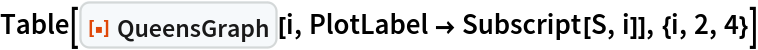Out=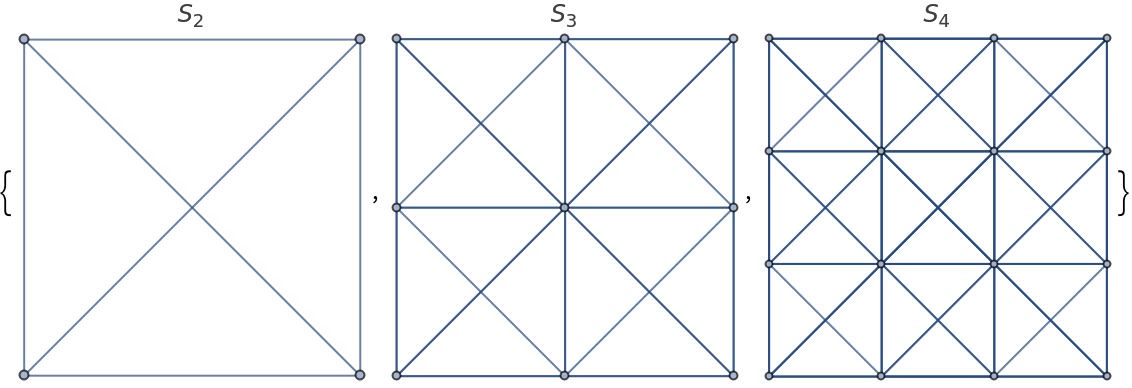Some rectangular queens graphs:

 In:=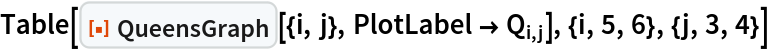Out=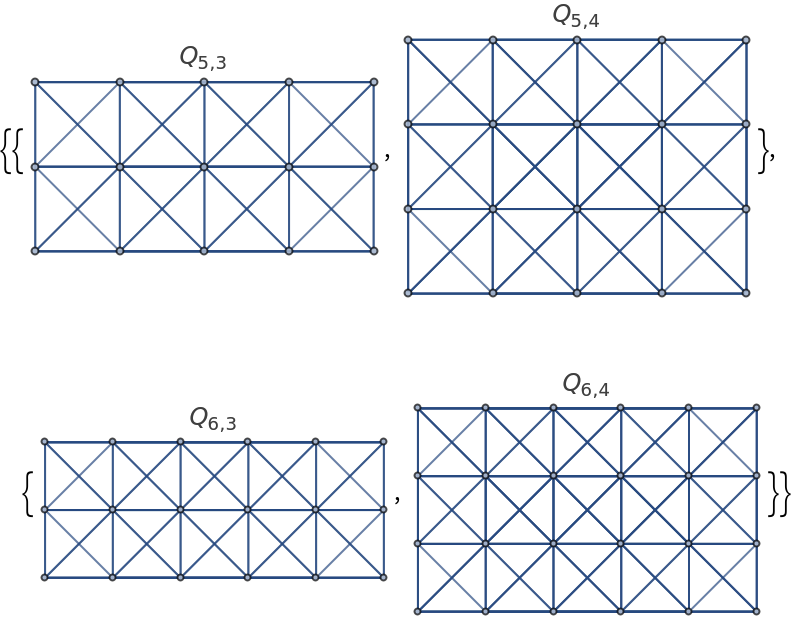### Scope (1)

The n-Queens graph is automatically laid out in the order of the appearance of the square numbering on the chessboard:

 In:=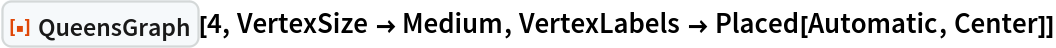Out=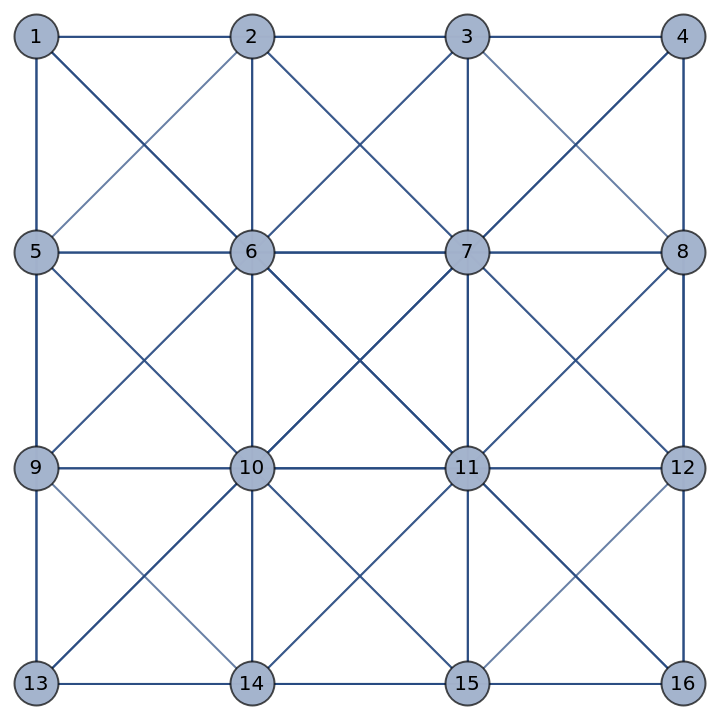### Options (6)

#### GraphHighlight (2)

Highlight vertex 1:

 In:=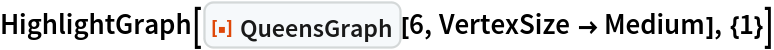Out=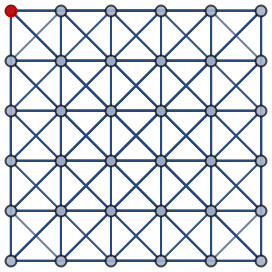Highlight the edge 26:

 In:=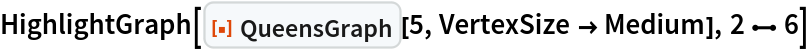Out=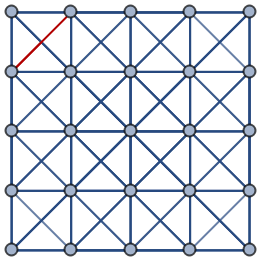#### VertexLabels (2)

Label individual vertices:

 In:=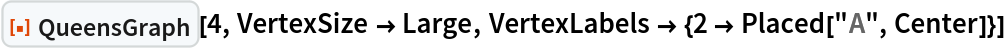Out=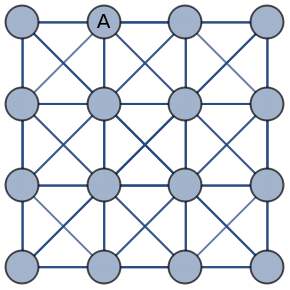Label all vertices:

 In:=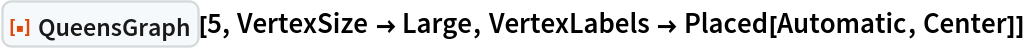Out=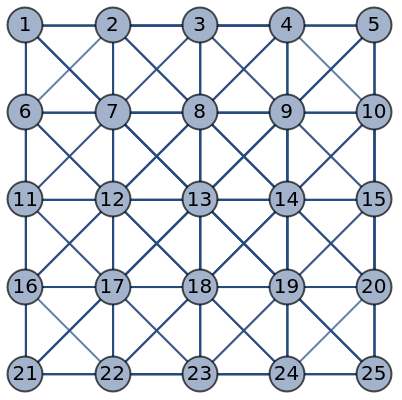#### VertexSize (2)

By default, the size of vertices is computed automatically:

 In:=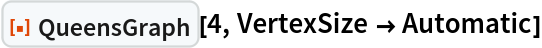Out=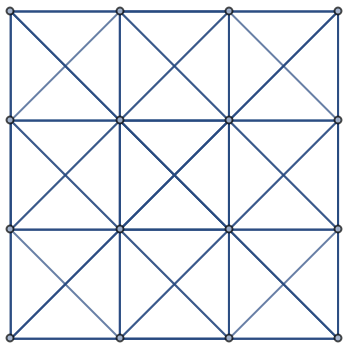Specify the size of all vertices using symbolic vertex size:

 In:=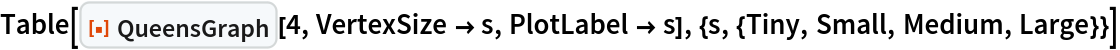Out=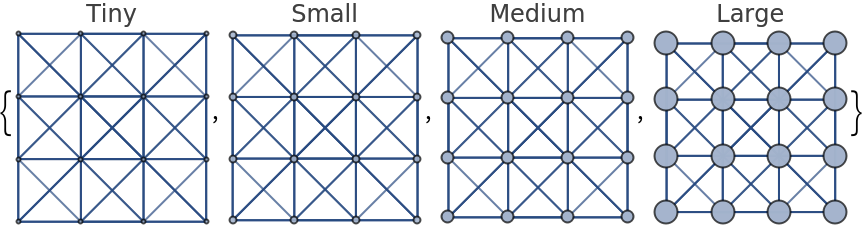### Applications (2)

Visualize valid moves for the queen:

 In:=Out=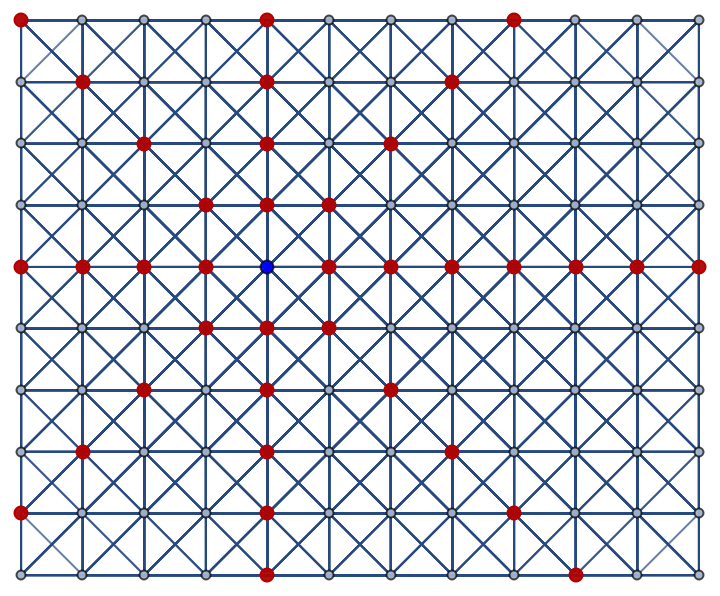The vertex coloring of the queen's graph:

 In:=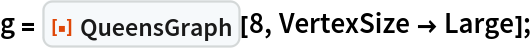In:=Out=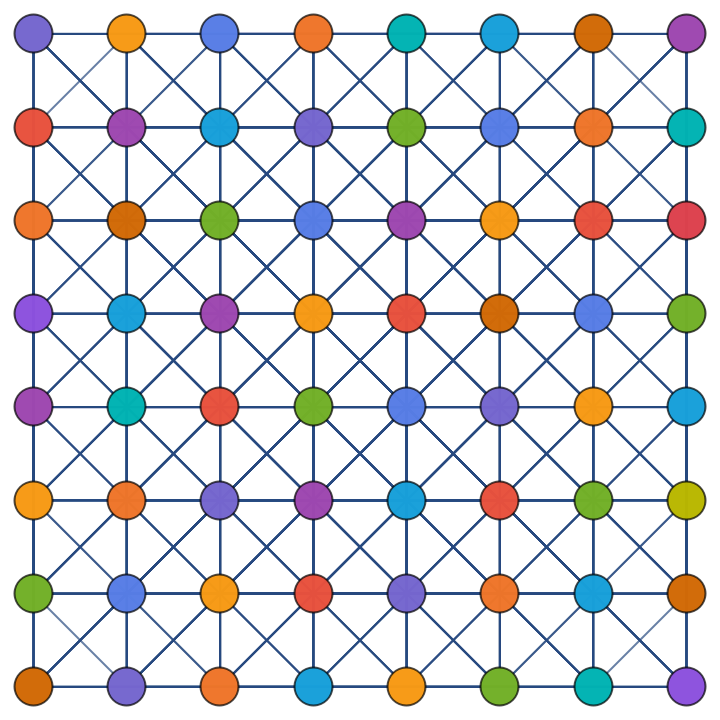## Publisher

Wolfram Summer School

## Version History

• 1.0.0 – 21 June 2023